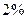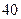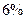Chapter 20, Problem 32P### Principles of Economics 2e

2nd Edition
Steven A. Greenlaw; David Shapiro
ISBN: 9781947172364

#### Solutions

Chapter
Section### Principles of Economics 2e

2nd Edition
Steven A. Greenlaw; David Shapiro
ISBN: 9781947172364
Textbook Problem

# An economy starts off with a GDP per capita ofHow large will the GDP per capita be if it grows at an annual rate offoryears?foryears?foryears?foryears?

To determine

GDP growth rate is to be determined.

Explanation

Given information:

GDP per capita= $5000 Growth rate= annual rate of 2% for 2 years, 2% for 40 years, 4% for 40 years, 6% for 40 years. Calculation: To calculate the answer, remember that: Starting Amount (1 + Growth Rate)Number of Years = Ending Amount So under the various scenarios in the question we have:$5,000(1 + .02)20 = $5,000(1.49) =$7,450$5,000(1 + .02)40 =$5,000(2

### Still sussing out bartleby?

Check out a sample textbook solution.

See a sample solution

#### The Solution to Your Study Problems

Bartleby provides explanations to thousands of textbook problems written by our experts, many with advanced degrees!

Get Started

#### Find more solutions based on key concepts